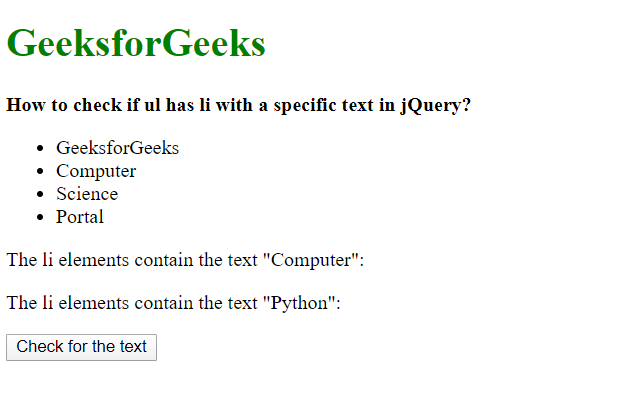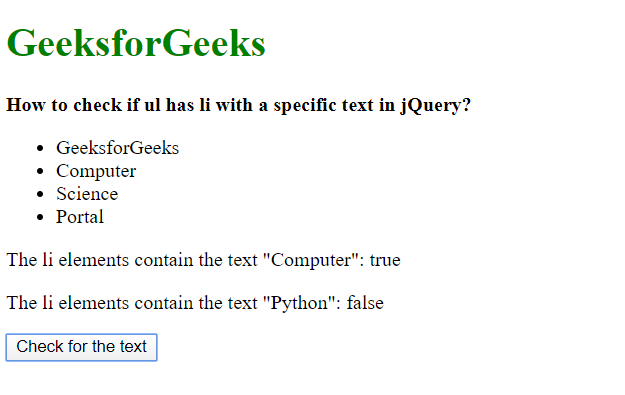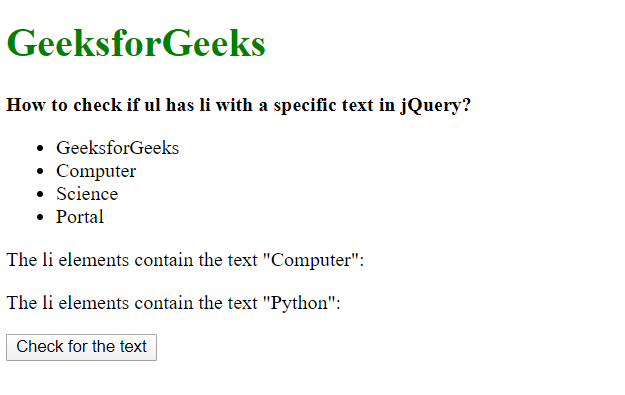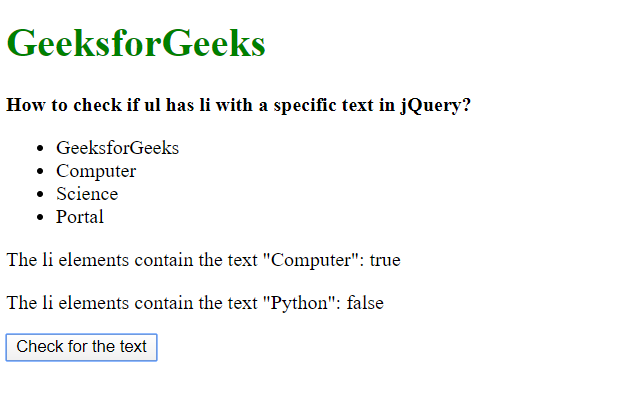# How to check if ul has li with the given text ?

Method 1: Using the each() method on the list to check the innerText property

Each of the list elements of the unordered list is first selected using a jQuery selector. The each() method is used on this list to iterate through it. This method has a callback function that returns the current index and the element of the iteration.

The text inside the returned element is checked for the innerText property to see if it matches the text required. A successful match means that the text inside the selected unordered list has the required text.

Syntax:

 `let found = ``false``; `   `\$(``"#list li"``).each((id, elem) => { ` `  ``if` `(elem.innerText == requiredText) { ` `    ``found = ``true``; ` `  ``} ` `}); `   `return` `found; `

Example:

 ` ` `<``html``> ` ` `  `<``head``> ` `    ``<``title``> ` `        ``Check if ul has li with a specific text in jQuery. ` `    `` ` ` ` ` `  `<``body``> ` `    ``<``h1` `style``=``"color: green"``> ` `    ``GeeksforGeeks ` `  `` ` `    ``<``b``> ` `    ``How to check if ul has li with ` `    ``a specific text in jQuery? ` `  `` ` `    ``<``ul` `id``=``"list"``> ` `        ``<``li``>GeeksforGeeks ` `        ``<``li``>Computer ` `        ``<``li``>Science ` `        ``<``li``>Portal ` `    `` ` `    ``<``p``> ` `        ``The li elements contain the text "Computer": ` `      ``<``span` `class``=``"output"``> ` `    `` ` `    `` ` `    ``<``p``> ` `        ``The li elements contain the text "Python": ` `      ``<``span` `class``=``"output2"``> ` `    `` ` `    `` ` `    ``<``button` `onclick``=``"runChecks()"``> ` `        ``Check for the text ` `    `` ` `    ``<``script` `src``=``"https://code.jquery.com/jquery-3.4.1.min.js"``> ` `    `` ` `    ``<``script``> ` `        ``function checkforText(requiredText) { ` `            ``let found = false; ` ` `  `            ``\$("#list li").each((id, elem) => { ` `                ``if (elem.innerText == requiredText) { ` `                    ``found = true; ` `                ``} ` `            ``}); ` ` `  `            ``return found; ` `        ``} ` ` `  `        ``function runChecks() { ` `            ``ans1 = checkforText('Computer'); ` `            ``document.querySelector(".output").textContent = ans1; ` ` `  `            ``ans2 = checkforText('Python'); ` `            ``document.querySelector(".output2").textContent = ans2; ` `        ``} ` `    `` ` ` ` ` `  ` `

Output:

• Before clicking the button:• After clicking the button:Method 2: Using the contains() selector
The contains() selector is used to select elements that have the text matching to the given one. This text could be present in any of the element’s descendants or the element itself. It takes one parameter that is the case-sensitive text to be matched.

The contains() selector is used along with the selector used for selecting the list elements. If no element is selected, that is, if the given text is not present, the number of elements returned would be 0. The number of returned elements could be checked with the length property and used to verify if the list contains the specified text.

Syntax:

 `let found = ``false``; `   `selector = ```#list :contains('\${requiredText}')` ` `selectedList = \$(selector); `   `if` `(selectedList.length) { ` `  ``found = ``true``; ` `} ` `return` `found; `

Example:

 ` ` `<``html``> ` ` `  `<``head``> ` `    ``<``title``> ` `        ``Check if ul has li with a specific text in jQuery. ` `    `` ` ` ` ` `  `<``body``> ` `    ``<``h1` `style``=``"color: green"``> ` `    ``GeeksforGeeks ` `  `` ` `    ``<``b``> ` `    ``How to check if ul has li with ` `    ``a specific text in jQuery? ` `  `` ` `    ``<``ul` `id``=``"list"``> ` `        ``<``li``>GeeksforGeeks ` `        ``<``li``>Computer ` `        ``<``li``>Science ` `        ``<``li``>Portal ` `    `` ` `    ``<``p``> ` `        ``The li elements contain the text "Computer": ` `      ``<``span` `class``=``"output"``> ` `    `` ` `    `` ` `    ``<``p``> ` `        ``The li elements contain the text "Python": ` `      ``<``span` `class``=``"output2"``> ` `    `` ` `    `` ` `    ``<``button` `onclick``=``"runChecks()"``> ` `        ``Check for the text ` `    `` ` `    ``<``script` `src``=``"https://code.jquery.com/jquery-3.4.1.min.js"``> ` `    `` ` `    ``<``script``> ` `        ``function checkforText(requiredText) { ` `            ``let found = false; ` ` `  `            ``selector ` `                ``= `#list :contains('\${requiredText}')` ` `            ``selectedList = \$(selector); ` ` `  `            ``if (selectedList.length) { ` `                ``found = true; ` `            ``} ` `            ``return found; ` `        ``} ` ` `  `        ``function runChecks() { ` `            ``ans1 = checkforText('Computer'); ` `            ``document.querySelector(".output").textContent = ans1; ` ` `  `            ``ans2 = checkforText('Python'); ` `            ``document.querySelector(".output2").textContent = ans2; ` `        ``} ` `    `` ` ` ` ` `  ` `

Output:

• Before clicking the button:• After clicking the button:My Personal Notes arrow_drop_upCheck out this Author's contributed articles.

If you like GeeksforGeeks and would like to contribute, you can also write an article using contribute.geeksforgeeks.org or mail your article to contribute@geeksforgeeks.org. See your article appearing on the GeeksforGeeks main page and help other Geeks.

Please Improve this article if you find anything incorrect by clicking on the "Improve Article" button below.

Article Tags :

Be the First to upvote.

Please write to us at contribute@geeksforgeeks.org to report any issue with the above content.